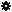﻿ Object Model: NurbsCurve.GetUFromNormalizedU

NurbsCurve.GetUFromNormalizedU

## NurbsCurve.GetUFromNormalizedU### Description

Returns the U actual value equivalent to the specified normalized U value.

### C# Syntax

 ```Double NurbsCurve.GetUFromNormalizedU( Double in_dUValueNormalized ); ```

### Scripting Syntax

 ```oDouble = NurbsCurve.GetUFromNormalizedU( UValueNormalized ); ```

### Parameters

Parameter Type Description
UValueNormalized Double The normalized UValue(0.0 to 1.0) from which we want the actual value equivalent.

### Examples

#### VBScript Example

 ```set oRoot = Application.ActiveProject.ActiveScene.Root set oArc = oRoot.AddGeometry( "Arc", "NurbsCurve" ) UValue = oArc.ActivePrimitive.Geometry.Curves(0).GetUFromNormalizedU( 0.7 ) LogMessage "The equivalent to the normalized U: 0.7 is U :" & UValue ```